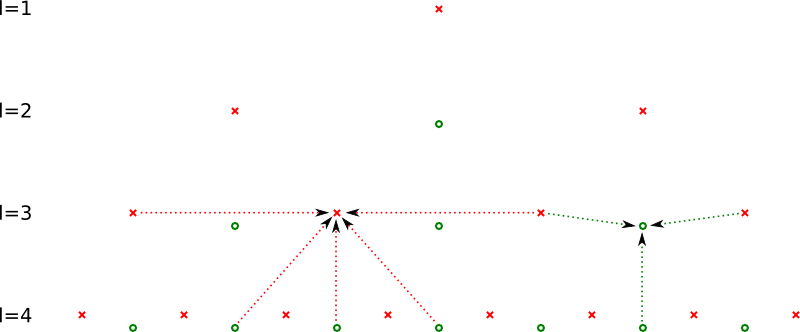SG++-Doxygen-Documentation
sgpp::base::ConvertLinearToPrewavelet Class Reference

Class that implements the transformation of a hierarchical linear sparse grid to a hierarchical prewavelet sparse grid. More...

#include <ConvertLinearToPrewavelet.hpp>

## Public Member Functions

Constructor, must be bind to a grid. More...

void operator() (DataVector &source, DataVector &result, grid_iterator &index, size_t dim)
Converts a given linear base to a prewavelet base. More...

~ConvertLinearToPrewavelet ()
Destructor. More...

## Protected Types

typedef GridStorage::grid_iterator grid_iterator

typedef index_t index_type

typedef level_t level_type

## Protected Attributes

GridStoragestorage
the grid object More...

## Detailed Description

Class that implements the transformation of a hierarchical linear sparse grid to a hierarchical prewavelet sparse grid.

Therefore the ()operator is implemented in order to use the sweep algorithm for the grid traversal. Let the coefficients from the hat basis be $$h_{l,i}$$ and from the prewavelet basis $$u_{l,i}$$. To calculate the surplusses, temp values are needed:

$(l,i)\neq G_{n}^{1}:t_{l,i}=-\frac{6}{10}u_{l,i\pm1}+t_{l+1,2i}$

All temp values for levels greater than the maximal level of the grid are set to 0. The actual transformation is defined by the following tridiagonal equation system:

\begin{eqnarray*} \frac{16}{10}u_{l,i}+\frac{4}{10}u_{l,i\pm2}&=&h_{l,i}-t_{l+1,2i}+\frac{1}{2}t_{l+1,2(i\pm1)}\\\frac{12}{10}u_{l,1}+\frac{4}{10}u_{l,3}&=&h_{l,1}-t_{l+1,2}+\frac{1}{2}t_{l+1,4}\\\frac{12}{10}u_{l,2^{l}-1}+\frac{4}{10}u_{l,2^{l}-3}&=&h_{l,2^{l}-1}-t_{l+1,2(2^{l}-1)}+\frac{1}{2}t_{l+1,2(2^{l}-2)} \end{eqnarray*}

For solving these tridiagonal systems, the method described in http://www.nrbook.com/a/bookcpdf/c2-4.pdf was used. Some odd Fileopen® plugin is needed to open that file ... sorry for that! the picture depicts all needed variables in oder to perform the transformation:This picture shows all involved gridpoints (red crosses) and temp values (green circles) to calculate the new hierarchical coefficients (red arrows) and new temp values (green arrows).

## ◆ grid_iterator

 protected

## ◆ index_type

 protected

## ◆ level_type

 protected

## ◆ ConvertLinearToPrewavelet()

 sgpp::base::ConvertLinearToPrewavelet::ConvertLinearToPrewavelet ( GridStorage & storage, GridStorage & shadowstorage )
inline

Constructor, must be bind to a grid.

An adaptive grid with prewavelet ansatz functions requires for operations using the up-down algorithm shadow points. These shadow points a needed just for data transport, thus they do not have an influence on the final function. Please refer to sgpp::pde::UpDownOneOpDimWithShadow for more information.

Parameters
 storage the grid storage object of the the grid, on which the hierarchisation should be executed shadowstorage shadow points (see detailed description)

## ◆ ~ConvertLinearToPrewavelet()

 sgpp::base::ConvertLinearToPrewavelet::~ConvertLinearToPrewavelet ( )
inline

Destructor.

References chess::dim, and operator()().

## ◆ operator()()

 void sgpp::base::ConvertLinearToPrewavelet::operator() ( DataVector & source, DataVector & result, grid_iterator & index, size_t dim )

Converts a given linear base to a prewavelet base.

Referenced by ~ConvertLinearToPrewavelet().